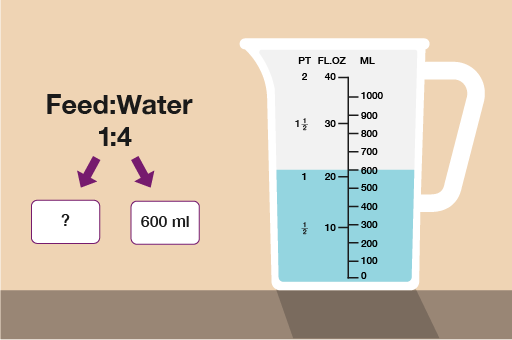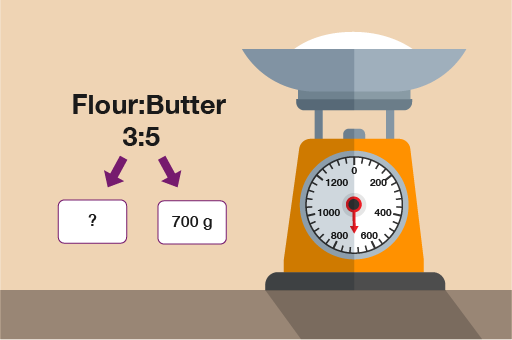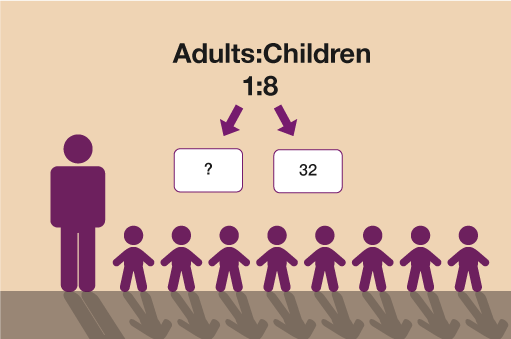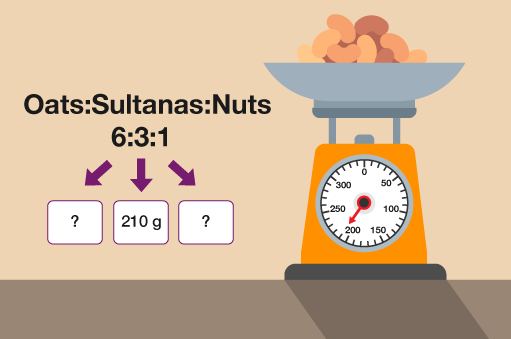Everyday maths 2 (Wales)

Start this free course now. Just create an account and sign in. Enrol and complete the course for a free statement of participation or digital badge if available.

# 10.3 Solving ratio problems where the total of one part of the ratio is given

Take a look at the worked example below:

You are growing tomatoes. The instructions on the tomato feed say:

• Use 1 part feed to 4 parts water

If you use 600 ml of water, how much tomato feed should you use?

These questions make much more sense if you look at them visually:Figure 15 Solving ratio problems to grow tomatoes

You can now see clearly that 600 ml of water is worth 4 parts of the ratio. To find one part of the ratio you need to do:

• 600 ml ÷ 4 = 150 ml

Since the feed is only 1 part, feed must be 150 ml. If feed was more than one part you would multiply 150 ml by the number of parts.

Just as with the previous type of question, you need to try to work out the value of 1 part. The value of any other number of parts can be worked out from this.

## Activity 26: Ratio problems with one part given

Practise your skills by tackling the ratio problems below:

1. A recipe requires flour and butter to be used in the ratio 3:5. The amount of butter used is 700 g.

How much flour will be needed?

1. Flour:ButterFigure 16 Using ratios in recipes

To find one part you do 700 g ÷ 5 = 140 g

To find the amount of flour needed you then do 140 g × 3 = 420 g flour.

1. When looking after children aged between 7 and 10, the ratio of adults to children must be 1:8.

• a.For a group of 32 children, how many adults must there be?
• b.If there was one more child in the group, how would this affect the number of adults required?

• a.Figure 17 Working out the ratio of adults to children

To find one part you do 32 ÷ 8 = 4.

• b.If there were 33 children, one part would be 33 ÷ 8 = 4.125.

Since you cannot have 4.125 adults, you need to round up to 5 adults so you would need one more adult for 33 children.

1. A shop mixes bags of muesli using oats, sultanas and nuts in the ratio 6:3:1.

If the amount of sultanas used is 210 g, how heavy will the bag of muesli be?

1. Oats:Sultanas:NutsFigure 18 Working out the ratio of oats, sultanas and nuts

Sultanas are 3 parts so to find 1 part you do 210 g ÷ 3 = 70 g.

Oats are 6 parts so 6 × 70 = 420 g.

Nuts are only 1 part so they are 70 g.

The total weight of the bag would be 210 g + 420 g + 70 g = 700 g.

Next you’ll look at ratio problems where only the difference in amounts is given.#### Find out more

*Eligibility rules apply for financial support.